In other words the genearl solution of eqn (5) is the linear combination of n linearly independent solutions.
on an open interval I where the functions pi (x) are continuous. Then, every solution of eqn (5) can be written as a linear combination of these solutions. That is, if y(x) is a solution then there are constants c1, c2, ....., cn such that

y(x) = c1 y1(x) + c2 y2 (x) + ..........+ cn yn (x) for all x in I
(5)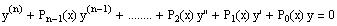Theorem: The general soltuion of Homogeneous Equations:

Let y1, y2, ...., y n be n linearly independent solutions of the the nth order homogenous equation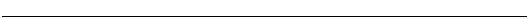Theorem3: (The wronskian of solutions)

See the text.
Definition: (The wronskian)

See the text.
Definition: (Hard to apply)

Two functions are linear indep if one is not a scalar multiple of the of the other.
Linearly independent functions
------------------------------------------------------------
This does not contradicts the theorem since dividing by x2 makes the coefficients of y` and y non continuous functions at x = 0 (where the initial values are specified.)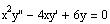are two solutions of the IVP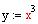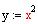Example (not working)
has a unique solution on the entire interval I.
y(n) + pn-1 y (n-1) + ......+ p1 y' + p0 y = f(x)

y(a) = b0 y1(a) = b2 y(n-1) (a) = bn-1
Existence and Uniqueness of solution to the IVP

Suppose the p0(x), p1(x), , pn-1 are cont on the open interval I containing the point a. The the IVP

for some constants c1,c 2, and c3.So the wronskian in never zero for all x. Therefore, solutions are linearly independent and the general solution is given by
Compute the Wronskian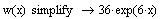Define the Wronskian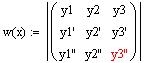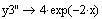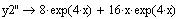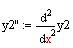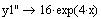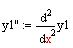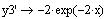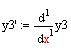Compute

the

derivatives

of

the

solutions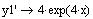Example

The function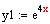,and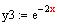are three solutions of the diff eqn

y(3) - 6 y'' +32 y =0 .

The wornskian of these solutions is
Example
Verify, by substation, that the two functions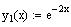and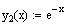are solutions to the differential equation y'' + 3y' + 2y =0
Note:
1. The principle of superposition is simply stating that a constant multiple of any solution is also a solution.

2. The sum of any number of solutions is also a solution.

3. The principle applies to homogenous linear diff eqns only.
The principle of superposition:

Let y1, y2, .., yn be n solutions of the homogeneous eqn (3) on an interval I, then the linear combination:

y = c1 y1 +.. + cn yn (for some constants c1, c2 , ...,cn )

is also a solution on I.
(3)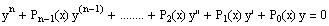Equation 2 is called the standard form of a nonhomogenous nth order linear differential equation. The associated homogeneous linear differential equation is given by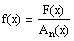and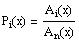where,
(2)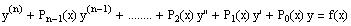then we can rewrite equation (1) as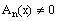If
Note:
1.denotes the nth derivative of y, that is2. Linearity is assured by requiring that the coefficient of the unknown function y and its derivatives be functions of x (and x alone).

3. The function F(x) is called the nonhomogenous term.

4. If F(x) = 0 then the differential equation is called the associated homogenous differential equation.
(1)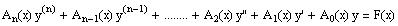The general nonhomogenous nth order linear differential equation has the form
The material of section 5.1 is limited to the 2nd order linear differential equations. The same topics are repeated in section 5.2 of the nth order linear differential equation. Thus, section 5.1 is a special case of section 5.2.
5.1-2-3 Higher Order Linear Diff Equations
Thus, the linear combination of the two solutions is also a solution.
Note: Mathcad is used to substitute the above values into the left-hand side of the diff eqn and it returned 0, which equals the right-hand side of the equation.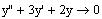(Substitute y and its derivatives into the differential equation.
(compute the 2nd deriv. of the first soln)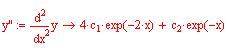(Compute the first deriv. of the first soln)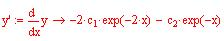(Define y as a lin. comb. of the two solns)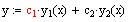Now, let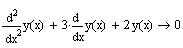Similarly, if, then (using mathcad to substitute in the eqn)
(substitute in the diff eqn)(compute the 2nd deriv. of the first soln)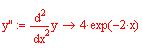(Compute the first deriv. of the first soln)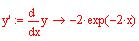(set y(x) equal the first son)